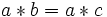# Groups are cancellative

## Statement

### Verbal statement

Any group is a cancellative monoid: every element in it is cancellative.

### Symbolic statement

Suppose$G$ is a group with binary operation$*$, and$a,b,c \in G$ are elements such that:$a * b = a * c$

then$b = c$

In other words$a$ is left cancellative. A similar proof shows that$a$ is right cancellative. In other words, given equations in terms of elements of the group, we can always cancel elements from the left and from the right.

## Proof

The proof follows from a somewhat more general fact: in a monoid (a set with associative binary operation and having identity element), any invertible element is cancellative: it can be canceled from the left or the right of any equation.